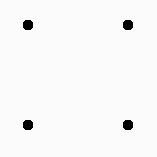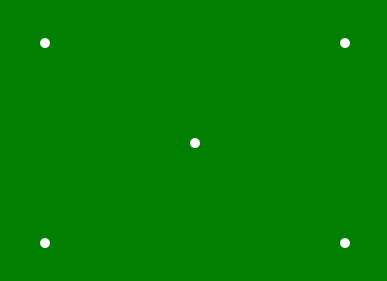Related Articles

# p5.js | point() Function

• Last Updated : 17 Jan, 2020

The point() function is an inbuilt function in p5.js which is used to draw the point in a given coordinate position.

Syntax:

`point( x, y, [z])`

Parameters: This function accept three parameters which are described below

• x: It is used to set the x-coordinate of point.
• y: It is used to set the y-coordinate of point.
• z: It is used to set the z-coordinate in WebGL mode.

Example 1:

 `function` `setup() {`` ` `    ``// Create Canvas of given size ``    ``createCanvas(400, 300);`` ` `}`` ` `function` `draw() {`` ` `    ``// Use point() function to draw point``    ``point(50, 50);``    ``point(150, 50);``    ``point(50, 150);``    ``point(150, 150);`` ` `    ``// Use strokeWeight() function to set``    ``// the weight of point``    ``strokeWeight(10);``}`

Output:Example 2:

 `function` `setup() { ``     ` `    ``// Create Canvas of given size ``    ``createCanvas(400, 300); ``} `` ` `function` `draw() { ``     ` `    ``// Set the background color``    ``background(``'green'``);``     ` `    ``// Set the stroke weight``    ``strokeWeight(10);`` ` `    ``// Set stroke color``    ``stroke(``'white'``);`` ` `    ``// Draw point``    ``point(50, 50);``   ` `    ``point(50, 250);``   ` `    ``point(350, 50);``   ` `    ``point(350, 250);``   ` `    ``point(200, 150);``   ` `} `

Output:Online editor: https://editor.p5js.org/
Environment Setup: https://www.geeksforgeeks.org/p5-js-soundfile-object-installation-and-methods/

Reference: https://p5js.org/reference/#/p5/point

My Personal Notes arrow_drop_up